×#### Thank you for registering.

One of our academic counsellors will contact you within 1 working day.

Click to Chat

1800-1023-196

+91-120-4616500

CART 0

• 0

MY CART (5)

Use Coupon: CART20 and get 20% off on all online Study Material

ITEM
DETAILS
MRP
DISCOUNT
FINAL PRICE
Total Price: Rs.

There are no items in this cart.
Continue Shopping• Complete JEE Main/Advanced Course and Test Series
• OFFERED PRICE: Rs. 15,900
• View Details

```Revision Notes on Comparing Quantities

Ratios

The ratio is used to compare two quantities. These quantities must have the same units.

The ratio is represented by “:”, which is read as “to”. We can write it in the form of “fraction”.Example

Write the ratio of the height of Sam to John, where Sam’s height is 175 cm and john’s height is 125 cm.

Solution

The ratio of Sam’s height to John’s height is 175: 125 = 7: 5.

We can write it in fraction as 7/5.

Equivalent Ratios

The equivalent ratio is like the equivalent fractions so to find the equivalent ratio we need to write it in the form of a fraction. To find the equivalent ratio we need to multiply or divide the numerator and denominator with the same number.

Example

Find the two equivalent ratios of 5: 20.

Solution

First multiply it by 2.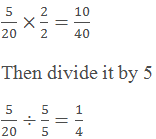So the two equivalent ratios are 10:40 and 1: 4.

To compare that the two ratios are equivalent or not we need to convert them in the form of like a fraction. Like fractions are the fractions with the same denominator.

Example

Check whether the ratios 2: 3 and 3: 4 are equivalent are not?

Solution

To check, first, we need to make their denominator same.Hence the ratio 2:3 is not equivalent to 3:4.

Proportion

Proportion shows the equality between two ratios. If two ratios are in proportion then these must be equal.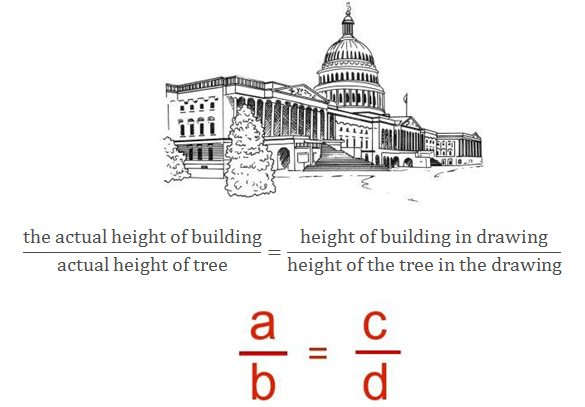How to solve proportion problems?

Example

If the cost of 8 strawberries is Rs. 64 then what will be the cost of 25 strawberries.Solution

Using Unitary Method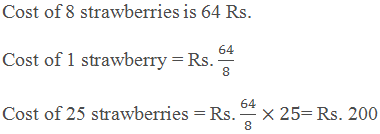Solution using proportion

Let the cost of 25 strawberries = Rs. x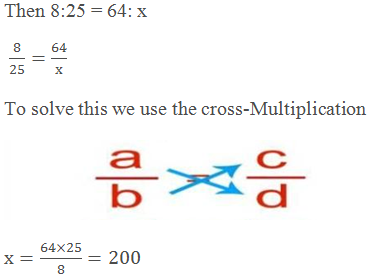Hence the cost of 25 strawberries is Rs. 200

Percentage

The percentage is another way of comparisons. In ratios we have to make the denominator same then only we can compare them but in percentage, we can compare by calculating the percentage of the given quantity.

The percentage is the numerator of the fraction with the 100 denominators.

Symbol of Percentage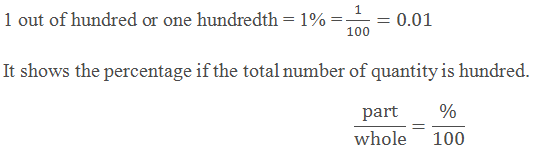Example

What is the percentage of boys and girls in the class of 100 students if the number of boys is 55 and the number of girls is 45?

Solution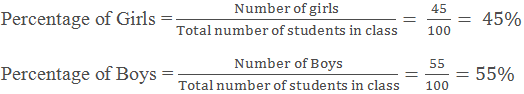Percentage if the total is not a hundred

If the total number of quantity is not hundred i.e. the denominator is not hundred then to find the percentage we need to make the denominator 100.

Example

Out of 4 bees, 2 are going right and 2 are going left. So what percentage of bees is going right?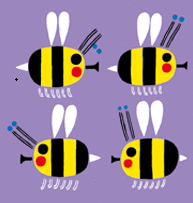Solution

Unitary Method

Out of 4 bees, the number of bees going right are 2. Hence, out of 100, the number of bees going right is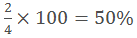By making denominator 100

Out of 4 bees, the number of bees going right is 2.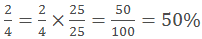Converting fractional numbers to percentage

Fractional numbers have different denominator and to convert them into percentage we have to multiply the fraction with the 100%.

Example

Out of 15 fishes, 5 are red. What is the percentage of the red fishes?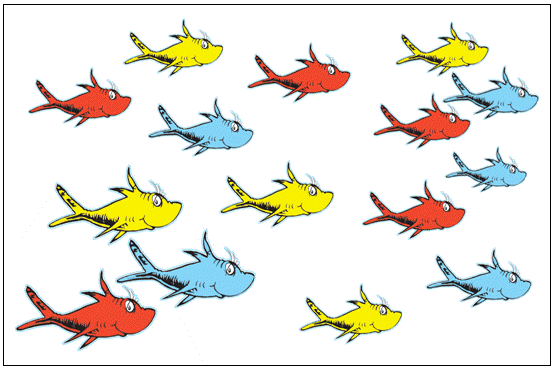Solution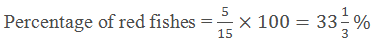Converting decimals to percentage

To convert the decimal into a percentage, first, we need to convert the decimal into fraction then multiply it by 100%.

Example

Convert 0.65 into a percentage.

Solution

Multiply the decimal with the 100%.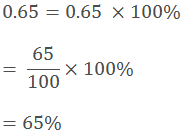Converting Percentage to fractions or Decimals

We can reverse the above process to convert the percentage into fraction or decimal.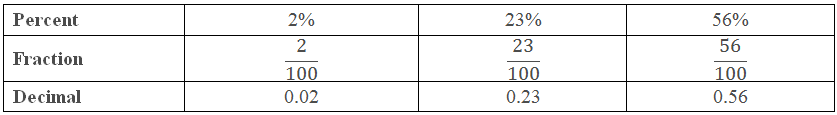Parts always add to give a whole

If we know the one part of a whole then we can find the other part because all the parts together form a whole or 100%.

Example

If there are 25 men in the office of 100 employees then the remaining 75 would be women.

This means that if 25% are men the (100% - 25%) = 75% are women.

Fun with estimation

With the help of percentage, we can estimate the parts of an area.

Example

What percent of the given figure is shaded?Solution

First, we have to find the fraction of the shaded portion.Use of Percentages

Interpreting percentages

To use the percentages in real life we must be able to interpret the percentage.

Example

If we say that Seema is spending 20% of her income then it means that Seema is spending Rs. 25 out of every Rs. 100 she earns.

Converting percentages to “How many”.

Example

If 20% of students get a distinction out of 45 students in a class, then how many students got the distinction?

Solution

The number of students got distinction = [20/100] × 45 = 9.

Hence, 9 students out of 45 got the distinction.

Ratios to percent

Example

If the profit of Rs. 2500 is divided among three partners in such a way that A, B and C got the two parts, three parts and five parts of profit respectively. How much money will each get? What percent of the profit do they get?

Solution

The three partners are getting profit in the ratio of 2: 3: 5, so the total of the parts is

2 + 3 + 5 = 10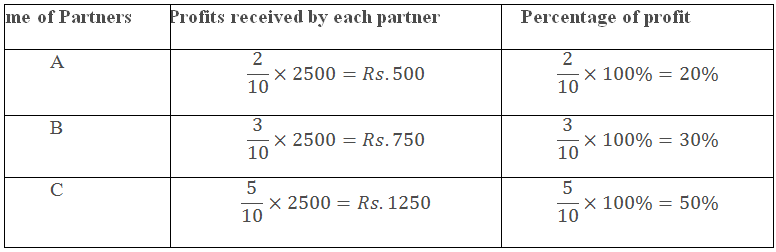Increase or decrease as Percent

Sometimes we have to find the increase or decrease in certain quantities as a percentage. Like the increase in population, decrease in sale etc.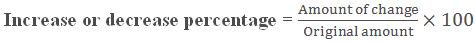Example

The total marks of Charlie increased from 365 to 380 from last year’s result. Find the increase in percentage.

Solution

Original amount = Marks of Charlie last year = 365

Amount of change = increase in the number of marks = 380 – 365 = 15.

Therefore,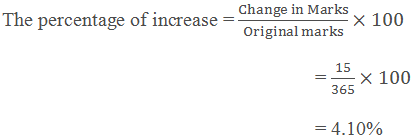Cost Price

Cost price is the price at which you buy some product. It is written as CP.

Selling Price

Selling price is the price at which you sell something. It is written as SP.

These are the factors which tell us that the sale of some product is profitable or not.

CP < SP

Profit

Profit = SP – CP

CP = SP

No profit no loss

-

CP > SP

Loss

Loss = CP - SP

Example

If the buying price (or CP) of a table is Rs 700 and the selling price (or SP) is Rs 820, then find the profit or loss.

Solution

As the SP is more than CP, so the seller earns the profit in the table.

Profit made = SP – CP

= Rs 820 – Rs 700

= Rs 80

Profit or loss percentage

The profit and loss can be converted into a percentage. It is always calculated on the cost price.Example

If the cost price of a laptop is Rs.45000 and the selling price is Rs. 50000, then what is the profit or loss percentage?

Solution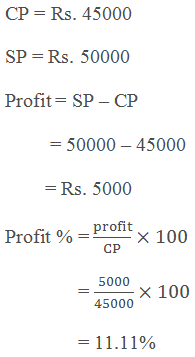How to find SP if CP and profit or loss % is given?Example

If the cost of a TV is Rs.25000 and shopkeeper sells it at a loss % of 5% then what is the selling price of the TV?

SolutionHence, the shopkeeper sells it at the price of Rs. 23750

How to find CP if SP and profit or loss % is given?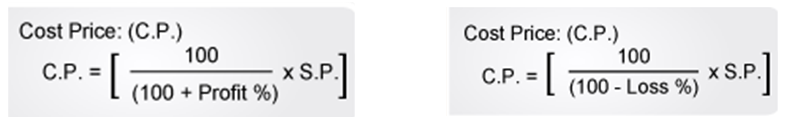Example

If the Selling price of a bookshelf is Rs 750 and the profit made by the seller is 10% then what is the cost price of the bookshelf?

Solution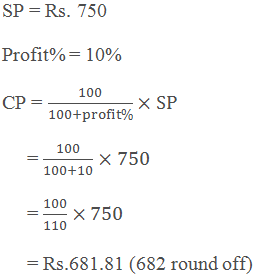Hence the seller bought the bookshelf at the cost of Rs. 682.

Simple Interest

When we borrow some money from the bank then we have to pay some interest to the bank.

The money which we borrow is called the Principal.

The amount which we have to pay to the bank to use that money is called interest.

At the end of the year we return the money to the bank with interest, that money is called Amount.

Amount = Principal + interest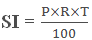Where,

SI = Simple interest

P = Principal

R = Rate of Interest

T = time period

Example

Sunita borrows a loan of Rs 5,0000 at 15% per year as the rate of interest. Find the interest she has to pay at end of one year.

Solution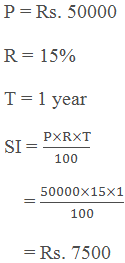Total amount to be paid by Sunita at the end of one year = Rs.50000 + Rs. 7500 = Rs.57500.

Interest for multiple years

If we have to calculate the interest for more than one year then we have to change the time period only.

Example

In the above example if Sunita takes the loan for 3 years then what will be the total amount after 3 years?

SolutionTotal amount to be paid by Sunita at the end of 3 years = Rs.50000 + Rs. 22500 = Rs.72500.
```### Course Features

• 728 Video Lectures
• Revision Notes
• Previous Year Papers
• Mind Map
• Study Planner
• NCERT Solutions
• Discussion Forum
• Test paper with Video Solution